﻿ 基于缩放因子和协作算子的肾脏算法 Kidney-Inspired Algorithm Based on Scaling Factor and Cooperative Operator

Computer Science and Application
Vol.08 No.04(2018), Article ID:24507,8 pages
10.12677/CSA.2018.84052

Kidney-Inspired Algorithm Based on Scaling Factor and Cooperative Operator

Qi Ke, Jiechang Wen

School of Applied Mathematics, Guangdong University of Technology, Guangzhou Guangdong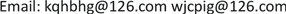Received: Apr. 5th, 2018; accepted: Apr. 18th, 2018; published: Apr. 25th, 2018ABSTRACT

In this paper, the kidney-inspired algorithm (KA) is studied in the optimization problem. It improves the defects in the kidney algorithm (KA) and shows good results in many optimization problems. For the KA algorithm, there is a problem that the search accuracy is low and it is easy to fall into the local optimum early on some functions. A cooperative operator was introduced to increase the diversity of the population and a scaling factor was added to the reabsorption update formula to achieve the goal of jumping out of the local optimal solution. Experimental comparison shows that the improved algorithm is an effective stable algorithm with higher accuracy and faster convergence speed.

Keywords:Optimization, Kidney-Inspired Algorithm, Cooperative Operator, Scaling Factor1. 引言

2. KA算法

2.1. 虚拟溶质的运动

${s}_{i+1}={s}_{i}+rand\left({s}_{best}-{s}_{i}\right)$ (1)

s表示KA的一个解(或生物肾脏中的溶质)。 ${s}_{i}$ 是第i次迭代中的个体。rand的值是介于0和给定数之间的随机数，而 ${s}_{best}$ 是在过去的迭代中被算法发现的最优个体。

2.2. 过滤

${f}_{r}=\alpha ×\frac{{\sum }_{i}^{p}f\left({x}_{i}\right)}{p}$ (2)

α的值是一个常数值的范围(0, 1)，在算法开始时设定。p是种群的大小。 $f\left({x}_{i}\right)$ 是第i代中求解的目标函数，在这个公式中可以看出，每次迭代的过滤速率依赖于种群中所有解的目标函数值。过滤速率以调节α来调整算法的收敛性。在每一次迭代中，目标函数的值越接近全局最优值，因此计算基于这些解的过滤速率，提高了FB中个体的质量。

2.3. 重吸收

2.4. 分泌和排泄

1) 初始化种群，用计算个体的目标函数值，将种群最优个体作为当前全局最优 ${s}_{best}$

2) 设置过滤率 ${f}_{r}$ ,。设置较差个体集W,较优个体集FB；设置迭代次数mumofite。

3) 个体进入过滤过程，如果该个体适应度值优于 ${f}_{r}$ ，则该个体没有过滤，进入FB；否则该个体过滤后进入W。

4) 如果一个个体过滤后进入W，通过重吸收算子更新。若更新后个体适应度值差于 ${f}_{r}$ ，则从W中移除，然后随机加入一个新个体代替；若更新后个体适应度值优于 ${f}_{r}$ ，则重吸收进入FB。但如果优于FB中的最差个体 ${S}_{worst}$ ,则将 ${S}_{worst}$ 分泌到W；如果差于FB中的 ${S}_{worst}$ ，也将重吸收到FB中的个体分泌入W。

5) 将FB中的个体排序，并更新 ${s}_{best}$ ；合并FB和W，更新过滤率，进行下一次迭代。

3. 基于缩放因子和协作算子的肾脏算法

3.1. 加入缩放因子的重吸收更新公式

${s}_{i+1}={c}_{1}{s}_{i}+{c}_{2}rand\left({s}_{best}-{s}_{i}\right)$ (3)

3.2. 协作算子

3.2.1. 算术交叉操作

 , (4)

${{S}^{″}}_{i}=\left(1-r\right)\cdot {S}_{i}+r\cdot {s}_{best}$ . (5)

3.2.2. 两点交叉算子

${{S}^{\prime }}_{i}=\left({s}_{i}^{1},{s}_{i}^{2},\cdots ,{s}_{j}^{a},{s}_{j}^{a+1},\cdots ,{s}_{j}^{b},\cdots ,{s}_{i}^{n}\right)$ (6)

${{S}^{″}}_{i}=\left({s}_{j}^{1},{s}_{j}^{2},\cdots ,{s}_{i}^{a},{s}_{i}^{a+1},\cdots ,{s}_{i}^{b},\cdots ,{s}_{j}^{n}\right)$ (7)

3.3. 改进后的算法步骤

1) 初始化种群，计算个体的目标函数值，将种群最优个体作为当前全局最优 ${s}_{best}$

2) 设置过滤率 ${f}_{r}$ ，设置较差个体集W，较优个体集 ；设置迭代次数mumofite。

3) 个体进入过滤过程，如果该个体适应度值优于 ${f}_{r}$ ，则该个体没有过滤，进入FB；否则该个体过滤后进入W。

4) 如果一个个体过滤后进入W，通过重吸收算子更新。若更新后个体适应度值差于 ${f}_{r}$ ，则从W中移除，然后随机加入一个新个体代替；若更新后个体适应度值优于 ${f}_{r}$ ，则重吸收进入FB。但如果优于FB中的最差个体 ${S}_{worst}$ ,则将分泌到W；如果差于FB中的 ${S}_{worst}$ ，也将重吸收到FB中的个体分泌入W。

5) 将FB中的个体排序，执行协作算子，并更新 ${s}_{best}$ ；合并FB和W，更新过滤率，进行下一次迭代。

4. 实验设置和结果分析

4.1. 实验设置

4.2. 实验结果比较分析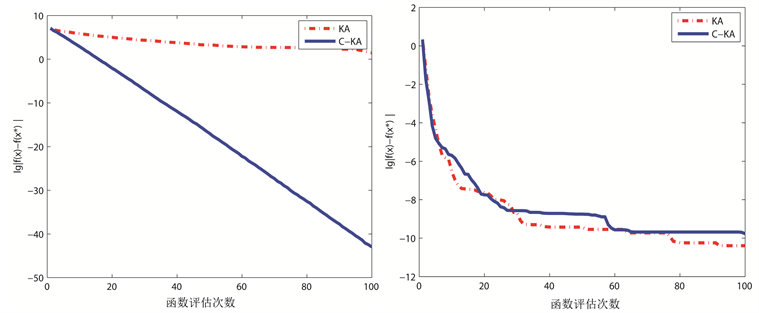Figure 1. Convergence curves of F1 and F2 functions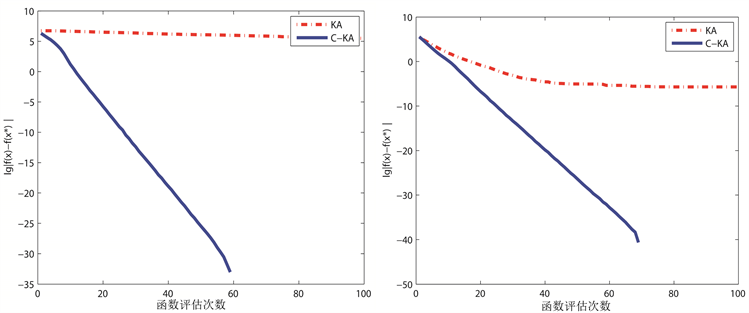Figure 2. Convergence curves of F3 and F4 functions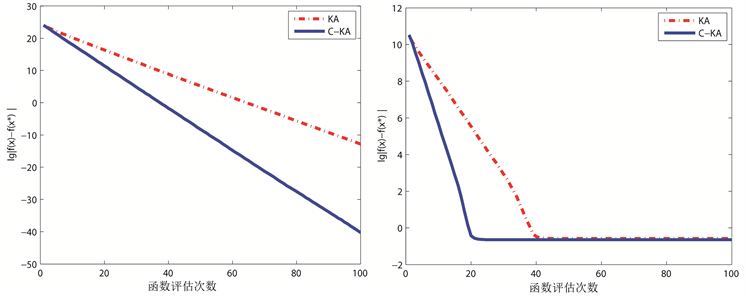Figure 3. Convergence curves of F5 and F6 functions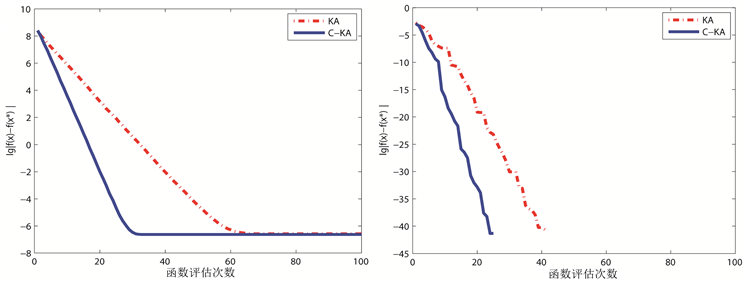Figure 4. Convergence curves of F7 and F8 functions

5. 结语

Kidney-Inspired Algorithm Based on Scaling Factor and Cooperative Operator[J]. 计算机科学与应用, 2018, 08(04): 472-479. https://doi.org/10.12677/CSA.2018.84052

1. 1. Jaddi, N.S., Alvankarian, J. and Abdullah, S. (2017) Kidney-Inspired Algorithm for Optimization Problems. Communi-cations in Nonlinear Science and Numerical Simulation, 42, 358-369. https://doi.org/10.1016/j.cnsns.2016.06.006

2. 2. 马红伟. 粒子群算法改进及其在数据挖掘中的应用研究[D]: [硕士学位论文]. 济南: 山东师范大学, 2014.

3. 3. Wang, H.C., Niu, D.X. and Chen, H.Y. (2017) Research on Coor-dination Planning of Micro Grid and Active Distribution Network Based on Kidney-Inspired Algorithm. Advances in Education Sciences, 12, 150-156.

4. 4. Liang, Y., Niu, D.X. and Wang, H.C. (2017) Assessment Analysis and Fore-casting for Security Early Warning of Energy Consumption Carbon Emissions in Hebei Province, China. Energies, 10, 391. https://doi.org/10.3390/en10030391

5. 5. 陈宝林. 最优化理论与算法[M]. 北京: 清华大学出版社, 2006.

6. 6. 金希东. 遗传算法及其应用[D]: [硕士学位论文]. 成都: 西南交通大学, 1996.

7. 7. 李士勇. 蚁群算法及其应用[M]. 哈尔滨工业大学出版社, 2004.

8. 8. 郭鹏. 差分进化算法改进研究[D]: [博士学位论文]. 天津: 天津大学, 2011.

9. 9. 徐焕芬, 刘伟, 谢月珊, 等. 双种群烟花算法[J]. 广东工业大学学报, 2017(5): 65-72.

10. 10. 张创业, 莫愿斌. 基于协同进化思想的人工鱼和粒子群混合优化算法[J]. 广西民族大学学报: 自然科学版, 2009, 15(1): 74-77.

11. 11. 慕彩红, 焦李成, 刘逸. M-精英协同进化数值优化算法[J]. 软件学报, 2009, 20(11): 2925-2938.

12. 12. 慕彩红, 焦李成, 刘逸. 求解约束优化问题M-精英协同进化算法[J]. 西安电子科技大学学报(自然科学版), 2010, 37(5): 854-861.

13. 13. 崔逊学. 多目标进化算法及其应用[M]. 北京: 国防工业出版社, 2006: 93-94.

14. 14. Liang, J., Suganthan, P.N. and Hernandez-Diaz, A.G. (2016) Problem Definitions and Evaluation Criteria for the CEC2013 Special Session on Real-Parameter Optimization.

15. 15. Liang, J.J., Qu, B.Y. and Suganthan, P.N. (2016) Problem Definitions and Evaluation Criteria for the CEC2014 Special Session and Competition on Single Objec-tive Real-Parameter Numerical Optimization. http://www.ntu.edu.sg/home/EPNSugan/index_files/CEC2014/CEC2014.htm

16. 16. 李荣雨, 周志勇. 成长性的粒子群算法及其在函数优化中的应用[J]. 信息与控制, 2017, 46(2): 224-230.

17. 17. 胡冠宇, 乔佩利. 混沌协方差矩阵自适应进化策略优化算法[J]. 吉林大学学报(工), 2017, 47(3): 937-943.